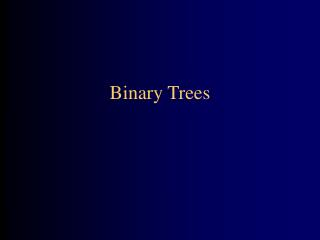DownloadDownload PresentationBinary Trees

# Binary Trees

Télécharger la présentation## Binary Trees

- - - - - - - - - - - - - - - - - - - - - - - - - - - E N D - - - - - - - - - - - - - - - - - - - - - - - - - - -
##### Presentation Transcript

1. Binary Trees

2. Linear data structures • Here are some of the data structures we have studied so far: • Arrays • Singly-linked lists and doubly-linked lists • Stacks, queues, and deques • Sets • These all have the property that their elements can be adequately displayed in a straight line • Binary trees are one of the simplest nonlinear data structures

3. Parts of a binary tree • A binary tree is composed of zero or more nodes • Each node contains: • A value (some sort of data item) • A reference or pointer to a left child (may be null), and • A reference or pointer to a right child (may be null) • A binary tree may be empty (contain no nodes) • If not empty, a binary tree has a root node • Every node in the binary tree is reachable from the root node by a unique path • A node with neither a left child nor a right child is called a leaf • In some binary trees, only the leaves contain a value

4. a b c d e g h i l f j k Picture of a binary tree

5. a b c d e f g h i j k l Size and depth • The size of a binary tree is the number of nodes in it • This tree has size 12 • The depth of a node is its distance from the root • a is at depth zero • e is at depth 2 • The depth of a binary tree is the depth of its deepest node • This tree has depth 4

6. a a b c b c e d e f g d f h i j g h A balanced binary tree i j An unbalanced binary tree Balance • A binary tree is balanced if every level above the lowest is “full” (contains 2n nodes) • In most applications, a reasonably balanced binary tree is desirable

7. Searching for 5:(0+6)/2 = 3 Using a binary search tree hi = 2;(0 + 2)/2 = 1 lo = 2;(2+2)/2=2 7 3 13 0 1 2 3 4 5 6 2 3 5 7 11 13 17 2 11 17 5 Binary search in an array • Look at array location (lo + hi)/2

8. A binary tree is defined recursively: it consists of a root, a left subtree, and a right subtree To traverse (or walk) the binary tree is to visit each node in the binary tree exactly once Tree traversals are naturally recursive Since a binary tree has three “parts,” there are six possible ways to traverse the binary tree: root, left, right left, root, right left, right, root root, right, left right, root, left right, left, root Tree traversals

9. Preorder traversal • In preorder, the root is visited first • Here’s a preorder traversal to print out all the elements in the binary tree: • public void preorderPrint(BinaryTree bt) { if (bt == null) return; System.out.println(bt.value); preorderPrint(bt.leftChild); preorderPrint(bt.rightChild);}

10. Inorder traversal • In inorder, the root is visited in the middle • Here’s an inorder traversal to print out all the elements in the binary tree: • public void inorderPrint(BinaryTree bt) { if (bt == null) return; inorderPrint(bt.leftChild); System.out.println(bt.value); inorderPrint(bt.rightChild);}

11. Postorder traversal • In postorder, the root is visited last • Here’s a postorder traversal to print out all the elements in the binary tree: • public void postorderPrint(BinaryTree bt) { if (bt == null) return; postorderPrint(bt.leftChild); postorderPrint(bt.rightChild); System.out.println(bt.value);}

12. The order in which the nodes are visited during a tree traversal can be easily determined by imagining there is a “flag” attached to each node, as follows: To traverse the tree, collect the flags: preorder inorder postorder A A A B C B C B C D E F G D E F G D E F G A B D E C F G D B E A F C G D E B F G C A Tree traversals using “flags”

13. Copying a binary tree • In postorder, the root is visited last • Here’s a postorder traversal to make a complete copy of a given binary tree: • public BinaryTree copyTree(BinaryTree bt) { if (bt == null) return null; BinaryTree left = copyTree(bt.leftChild); BinaryTree right = copyTree(bt.rightChild); return new BinaryTree(bt.value, left, right);}

14. Other traversals • The other traversals are the reverse of these three standard ones • That is, the right subtree is traversed before the left subtree is traversed • Reverse preorder: root, right subtree, left subtree • Reverse inorder: right subtree, root, left subtree • Reverse postorder: right subtree, left subtree, root

15. The End